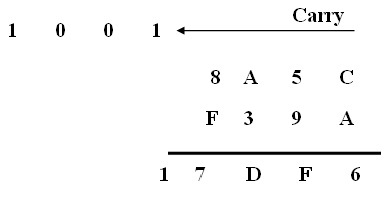Enter Hex Number (A) Enter Hex Number (B)
 Result: A+B in Hex A+B in Decimal

The Hex Numbers Addition Calculator is very easy to use. When you enter two hex numbers you will get the addition result in Hex and in equivalent Decimal number.

For example: The two hex numbers you enter are (8A5C)16 and (F39A)16

The Fig below show how to calculateThe hexadecimal number is (17DF6)16, the equivalent decimal result is (97782)10

Thinkcalculator.com provides you helpful and handy calculator resources.